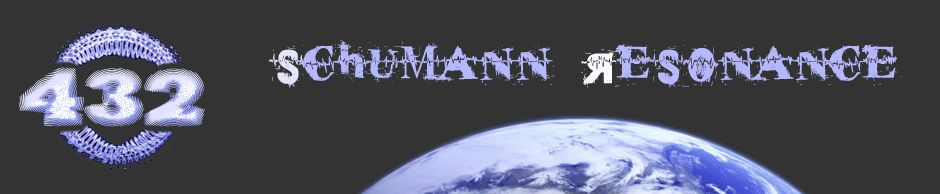# Blog » Myth ⚠ 432Hz & The Speed of Light

Last Updated on

Approx. reading time: 4 minutesNovember 20, 2013

## MYTH ⚠ 432Hz & THE SPEED OF LIGHT

“432 IS THE SQUARE ROOT (√) OF THE “SPEED OF LIGHT”.
(PSEUDOSCIENCE)

Some people like to suggest that 432Hz is the most natural concert pitch due to a presumed relation to the speed of light:

“432 is the square root of the Speed of Light”.

186624 = 432

The calculation above is correct … but does NOT make the statement right.

The actual speed of light is not exactly 186624, but (depending on the source) 299792458 meters/s. = 186282.397 miles/s. That is about 341.603 miles per second off. If you would use that number instead to calculate the square root, you get:

√186282.397 431,60444506515453634375

Some sources (nasa.gov) mention 186000 miles/s. If we use this number to calculate the square root:

186000 431,277173057

In both examples above we speak about the Speed of Light in a vacuum.

The difference between 432 and 431.6 or 431.2 might look insignificant, but I am pretty sure a math or physics teacher would not except “432” as answer, if the “assignment” was to calculate the square root of the Speed of Light (186282.397 or 186000 miles/s).

Besides that, only if we use miles as unit of length for the Speed of Light, we come close to 432 when calculating the square root. Usage of the mile as an official unit of measurement is largely confined to the United Kingdom, the United States, and Canada. If we would use other units, like meters (√299792458 = 17314,51581766) or yards (√327857018.81 = 18106,822438241), or other miles, we would not end up with ≈432 as the square root.

##### USING UNITS …

Another thing we can say about this theory, is that – if you want to compare or “relate” two “things” with one another – you have to use values that work for/with both ‘things’.

But we are not!

We are trying to relate the unit Hz (a particular number of occurrences of a repeating event per unit time) to the unit of speed “miles per second” (the magnitude of the velocity – the rate of change of its position

After all, we are not talking about 186282.397Hz, but 186282.397 miles/s. when we speak about the Speed of Light.

The unit “speed” and “Hz” can not simple be related, compared or exchanged 1 on 1 by one another!##### CONDITIONS & DIFFERENCES SOUND AND LIGHT WAVES

Only when electromagnetic waves (radio, microwave, infrared, the visible spectrum we perceive as: visible light, ultraviolet, X-rays, and/or gamma rays) propagate* through a VACUUM, the electromagnetic waves “propagates” with the Speed of Light, at 186282.397 miles/second. Under other conditions (through a medium) light propagates slower.

* Some scientist, theorists and natural philosophers such as Walter Russell state that waves of light do not propagate but ‘reproduce’ each other from wave field to wave field of space. The planes of zero curvature, which bound all wave fields, act as mirrors to reflect light from one field into another. This sets up an appearance of light as traveling, which is pure illusion.

The properties of a sound wave always depend on the properties of the medium it travels through: no medium (vacuum) = no Sound. The speed of sound in air (c) = 343 meters/s at 20°C. The speed of sound through air could vary slightly, depending on the temperature, pressure and humidity. The speed of sound in water is about 4 times faster than this.

Do you see the first “problem” we run into?

We practically try to relate a particular frequency through a medium (air) to the  Speed of Light (186282.397 m/s.) in a vacuum (= no medium) in which the speed of sound waves would always be “0” (nonexistent)!!!

OTHER DIFFERENCES:

• The frequencies of visible light and audible sound differ from each other by more than ten orders of magnitude. Audible acoustic range: roughly 20 Hz to 20,000 Hz vs. visible optical range: roughly 380 trillion Hz to 760 trillion Hz.
• Light waves are composed of transverse waves in an electromagnetic field, while sound waves are mechanical longitudinal waves (alternate compression and expansion of matter).
• The denser the medium, the greater the speed of sound. The opposite is true of light.
• The speed of light in a medium is constant. The velocity of sound waves can change.
• Electromagnetic waves, including light is a “stream of particles” (photons). Sound does not consist out of particles.
• Light waves can be polarized, but sound waves cannot.

##### CONCLUSION?

When simply looking at the numbers “432” and “186624” (186282.397) without proper context, one could easily draw wrong conclusions.

No matter how we look at it, there is no real direct relationship between the tone / frequency of 432Hz and the Speed of Light. The “432Hz & the Speed of Light” story is a pseudo-scientific fantasy.

##### IS THERE NO OTHER RELATIONSHIP BETWEEN SOUND AND LIGHT?

The answer to this question is … yes there is! 🙂
You can read more about it in blog articles: “Sound & Light (colour)” and “Sound (256Hz & 432Hz) and Colors“.

REFERENCES:

NOTE: The wavelenght Calculator linked to above, uses meters/s not miles/s, so you first have to convert miles to meters.